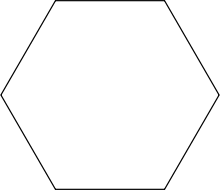## How to Calculate and Solve for the Length of a Side and Perimeter of a Hexagon | The Calculator EncyclopediaThe image above is a hexagon.

To compute the perimeter of a hexagon, one essential parameter is needed and this parameter is length of side (a).

The formula for calculating the perimeter of a pentagon:

P = 6a

Where;
P = Perimeter of the hexagon
a = length of side

Let’s solve an example;
Find the perimeter of a hexagon when the length of side is 15 cm.

This implies that;
a = length of side = 15 cm

P = 6a
P = 6 x 15
P = 90

Therefore, the perimeter of the hexagon is 90 cm.

Calculating the length of side (a) using the Perimeter of the hexagon.

a = P / 6

Where;
a = length of side
P = Perimeter of the hexagon

Let’s solve an example;
Find the length of side when the perimeter of the hexagon is 120 cm.

This implies that;
P = Perimeter of the hexagon = 120 cm

a = P / 6
a = 120 / 6
a = 20

Therefore, the length of side (a) is 20 cm.

## How to Calculate and Solve for the Length of a Side and Area of a Hexagon | The Calculator EncyclopediaThe image above is a hexagon.

To compute the area of a hexagon, one essential parameter is needed and this parameter is length of side (a).

The formula for calculating the area of a hexagon:

A = (a2)3√32

Where;
A = Area of the hexagon
a = Length of side

Let’s solve an example;
Find the area of a hexagon when the length of side is 35 cm.

A = (a2)3√32
A = (352)3√32
A = 1225 x 3(1.73)2
A = 1225 x 5.1962
A = 6365.2872
A = 3182.64

Therefore, the area of the hexagon is 3182.64 cm2.

Calculating the length of side (a) using the area of the hexagon.

a = √(2A3√3)

Where;
a = length of side
A = Area of the hexagon

Let’s solve an example;
Given that the area of the hexagon is 120 cm2. Find the length of side?

This implies that;
A = Area of the hexagon = 120 cm2

a = √(2A3√3)
a = √(2 x 1205.196)
a = √(2405.196)
a = √46.189
a = 6.796

Therefore, the length of side (a) is 6.796 cm.